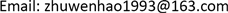1. 引言

2. 同步发电机的数学模型

1) 忽略定子磁暂态过程；

2) 计及转子d轴、q轴的暂态过程及次暂态过程。

d轴模型

{ T ′ d 0 ⋅ d E ′ q d t = E f − E ′ q − x d − x ′ d x ′ d − x ″ d ( E ′ q − E ″ q ) T ″ d 0 ⋅ d E ″ q d t = E ′ q − E ″ q − ( x ′ d − x ″ d ) i d + T ″ d 0 ⋅ d E ′ q d t u q = E ″ q − x ″ d i d E f = k ⋅ u f d (1)

q轴模型

{ T ′ q 0 ⋅ d E ′ d d t = − E ′ d − x q − x ′ q x ′ q − x ″ q ( E ′ d − E ″ d ) T ″ q 0 ⋅ d E ″ d d t = E ′ d − E ″ d + ( x ′ q − x ″ q ) i q + T ″ q 0 ⋅ d E ′ d d t u d = E ″ d + x ″ q i q (2)

3. 发电机参数辨识

x q = U d / I q (3)

x d = x q (4)

3.1. 目标函数

J d = ∑ t = 1 n ( i dc − i dm ) 2 (5)

J q = ∑ t = 1 n ( i qc − i qm ) 2 (6)

3.2. 微分方程的求解

y ¯ n + 1 = y n + h f ( x n , y n ) (7)

y n + 1 = y n + h 2 [ f ( x n , y n ) + f ( x n + 1 , y ¯ n + 1 ) ] (8)

4. 优化算法

5. 算例分析验证

Comparison of identification result-direct axi

PSO4.87 × 10−40.2990.12308.9340.071
AGA5.28 × 10−50.2570.13208.2340.041
PSO误差%35.90−35.268.9591.89
AGA误差%16.8−30.50.4110.81

PSO5.24 × 1040.2970.2521.3820.137
AGA3.24 × 1050.3510.2021.1920.075
PSO误差%−21.8432.6338.20204.04
AGA误差%−7.636.3119.2066.67

6. 结论